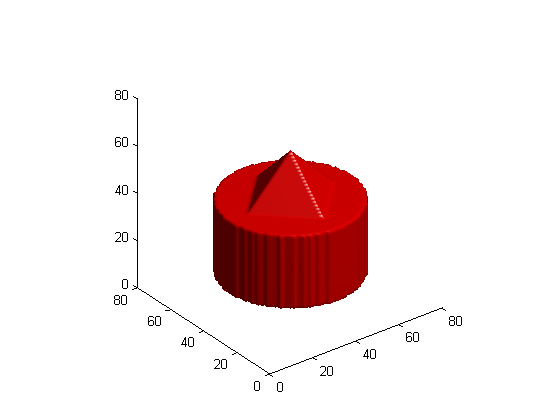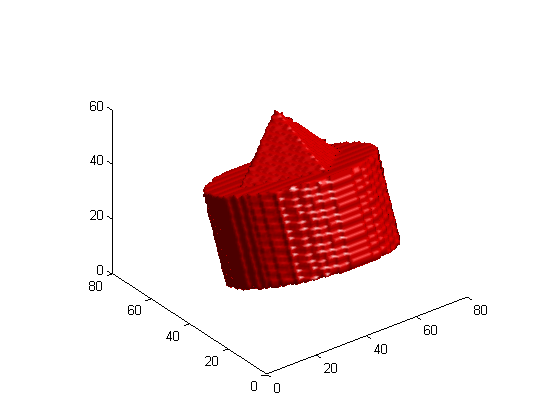# Spatial transformations: Three-dimensional rotation

Blog reader Stephen N., who's been following my posts about spatial transformations, asked me last week how to rotate a three-dimensional image.

The Image Processing Toolbox function tformarray is a very general multidimensional spatial transformer and can be used for three-dimensional rotation. Here's how.

### Make a three-dimensional blob

First, let's make a three-dimensional image containing a blob that will easily show the effect of a rotation.

[x,y,z] = ndgrid(-1:.025:1);
blob = z <= 0 & z >= -0.75 & x.^2 + y.^2 <= sqrt(0.25);
blob = blob | (z > 0 & (abs(x) + abs(y) <= (0.5 - z)));

Display the blob using isosurface and patch.

p = patch(isosurface(blob,0.5));
set(p, 'FaceColor', 'red', 'EdgeColor', 'none');
daspect([1 1 1]);
view(3)
camlight
lighting gouraud### Make a 3-D affine tform struct

We want to rotate the blob about its own center. For me, the simplest way to construct an affine transform matrix that will do that is to use three steps:

1. Translate the middle of the blob to the origin.

2. Rotate the blob.

3. Translate the rotated blob back to its starting location.

Here's the first translation:

blob_center = (size(blob) + 1) / 2
blob_center =

41    41    41


T1 = [1 0 0 0
0 1 0 0
0 0 1 0
-blob_center 1]
T1 =

1     0     0     0
0     1     0     0
0     0     1     0
-41   -41   -41     1



Now here's the rotation. In this example we'll rotate about the second dimension.

theta = pi/8;
T2 = [cos(theta)  0      -sin(theta)   0
0             1              0     0
sin(theta)    0       cos(theta)   0
0             0              0     1]
T2 =

0.9239         0   -0.3827         0
0    1.0000         0         0
0.3827         0    0.9239         0
0         0         0    1.0000



And here's the final translation.

T3 = [1 0 0 0
0 1 0 0
0 0 1 0
blob_center 1]
T3 =

1     0     0     0
0     1     0     0
0     0     1     0
41    41    41     1



The forward mapping is the composition of T1, T2, and T3.

T = T1 * T2 * T3
T =

0.9239         0   -0.3827         0
0    1.0000         0         0
0.3827         0    0.9239         0
-12.5691         0   18.8110    1.0000


tform = maketform('affine', T);

Let's do a quick sanity check: the tform struct should map the blob center to itself.

tformfwd(blob_center, tform)
ans =

41    41    41



### What the tformarray inputs mean

Now let's see how to make the inputs to the tformarray function. The syntax of tformarray is B = tformarray(A, T, R, TDIMS_A, TDIMS_B, TSIZE_B, TMAP_B, F).

A is the input array, and T is the tform struct.

R is a resampler struct produced by the makeresampler function. You tell makeresampler the type of interpolation you want, as well as how to handle array boundaries.

R = makeresampler('linear', 'fill');

TDIMS_A specifies how the dimensions of the input array correspond to the dimensions of the spatial transformation represented by the tform struct. Here I'll use the simplest form, in which each spatial transformation dimension corresponds to the same input array dimension. (Don't worry about the details here. One of these days I'll write a blog posting showing an example of when you might want to do something different with this dimension mapping.)

TDIMS_A = [1 2 3];

TDIMS_B specifies how the dimensions of the output array correspond to the dimensions of the spatial transformation.

TDIMS_B = [1 2 3];

TSIZE_B is the size of the output array.

TSIZE_B = size(blob);

TMAP_B is unused when you have a tform struct. Just specify it to be empty.

TMAP_B = [];

F specifies the values to use outside the boundaries of the input array.

F = 0;

### Call tformarray to transform the blob

blob2 = tformarray(blob, tform, R, TDIMS_A, TDIMS_B, TSIZE_B, TMAP_B, F);

### Display the rotated blob

clf
p = patch(isosurface(blob2,0.5));
set(p, 'FaceColor', 'red', 'EdgeColor', 'none');
daspect([1 1 1]);
view(3)
camlight
lighting gouraudPublished with MATLAB® 7.2

|

### 댓글

댓글을 남기려면 링크 를 클릭하여 MathWorks 계정에 로그인하거나 계정을 새로 만드십시오.